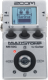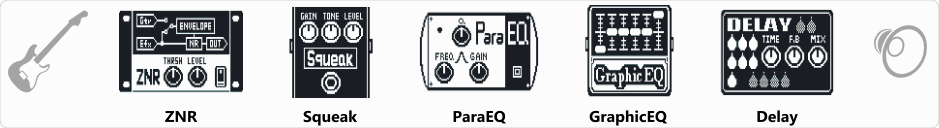# RAT Drive

Discussion in 'Zoom MS-50G' started by Tonelib, May 20, 2018.

1. RAT DriveDevice: Zoom Ms-50g
Firmware: 3.00

Name on device: RAT Drive
Optimized for: Guitar Amp

Effects chain:Factory Zoom MS-50G preset 

Effect: "ZNR" (Dynamics / Filter), active - "yes"
"THRSH" = 1
"DETCT" = EfxIn
"Level" = 100

Effect: "Squeak" (Overdrive / Distortion), active - "yes"
"Gain" = 49
"Tone" = 25
"Level" = 100

Effect: "ParaEQ" (Dynamics / Filter), active - "yes"
"Freq1" = 630Hz
"Q1" = 1
"Gain1" = 2
"Freq2" = 630Hz
"Q2" = 1
"Gain2" = 0
"Level" = 100

Effect: "GraphicEQ" (Dynamics / Filter), active - "yes"
"160Hz" = 0
"400Hz" = -2
"800Hz" = -1
"3.2kHz" = 1
"6.4kHz" = -3
"12kHz" = -1
"Level" = 100

Effect: "Delay" (Delay / Reverb), active - "yes"
"Time" = 421
"F.B" = 16
"Mix" = 26
"HiDMP" = 10
"P-P" = MONO
"Level" = 100
"Tail" = On

Note: This is a patch file, you will need to download and install the ToneLib software to use the patch.

File size:
409 bytes
Views:
422